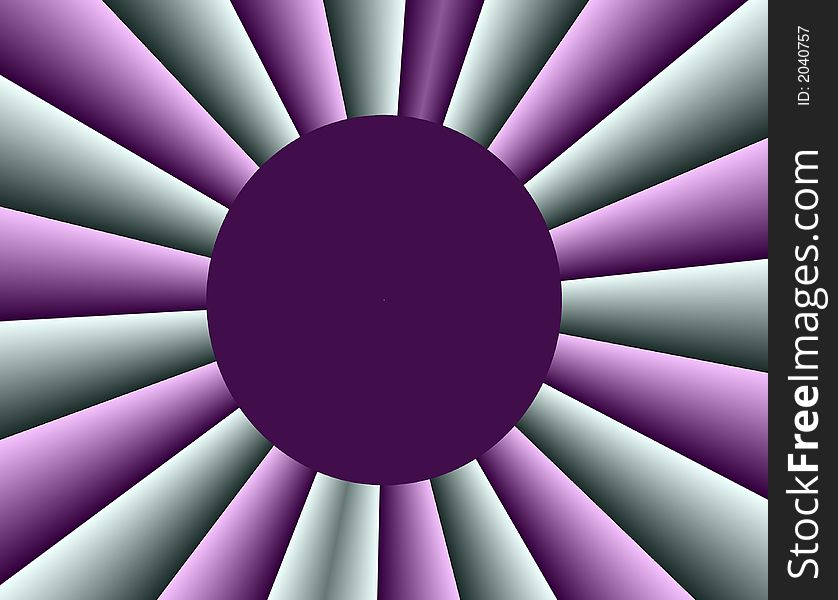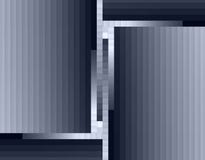Stock Image Of Fractal Geometry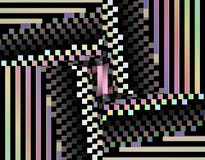Stock Image Of Fractal Geometry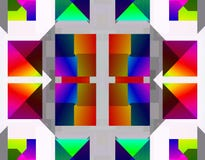Stock Image Of Fractal Geometry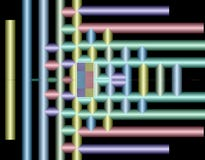Stock Image Of Fractal Geometry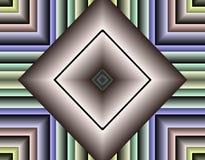Stock Image Of Fractal Geometry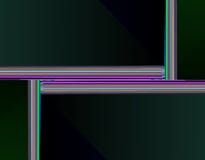Stock Image Of Fractal Geometry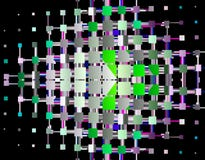Stock Image Of Fractal Geometry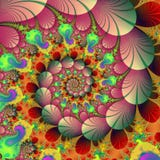Stock Image Of Fractal Autumn Background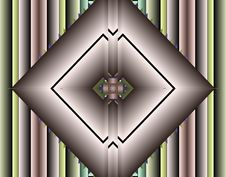Stock Image Of Fractal Geometry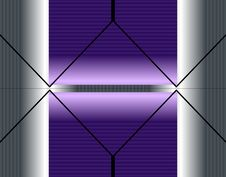Stock Image Of Fractal Geometry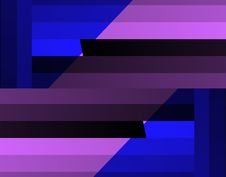Stock Image Of Fractal Geometry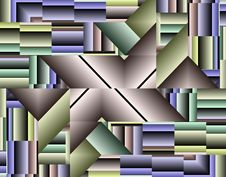Stock Image Of Fractal Geometry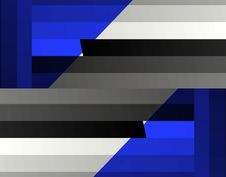Stock Image Of Fractal GeometryStock Image Of Fractal Geometry